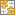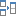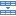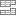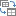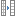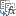# Excel SEQUENCE function - create a number series automatically

In this tutorial, you will learn how to create a number sequence in Excel with formulas. Additionally, we'll show you how to auto generate a series of Roman numbers and random integers - all by using a new dynamic array SEQUENCE function.

The times when you had to put numbers in sequence in Excel manually are long gone. In modern Excel, you can make a simple number series in a flash with the Auto Fill feature. If you have a more specific task in mind, then use the SEQUENCE function, which is specially designed for this purpose.

## Excel SEQUENCE function

The SEQUENCE function in Excel is used to generate an array of sequential numbers such as 1, 2, 3, etc.

It is a new dynamic array function introduced in Microsoft Excel 365. The result is a dynamic array that "spills" into the specified number of rows and columns automatically.

The function has the following syntax:

SEQUENCE(rows, [columns], [start], [step])

Where:

Rows (optional) - the number of rows to fill.

Columns (optional) - the number of columns to fill. If omitted, defaults to 1 column.

Start (optional) - the starting number in the sequence. If omitted, defaults to 1.

Step (optional) - the increment for each subsequent value in the sequence. It can be positive or negative.

• If positive, subsequent values increase, creating an ascending sequence.
• If negative, subsequent values decrease, producing a descending sequence.
• If omitted, the step defaults to 1.

## Basic formula to create a number sequence in Excel

If you are looking to populate a column of rows with sequential numbers starting at 1, you can use the Excel SEQUENCE function in its simplest form:

To put numbers in a column:

SEQUENCE(n)

To place numbers in a row:

SEQUENCE(1, n)

Where n is the number of elements in the sequence.

For example, to populate a column with 10 incremental numbers, type the below formula in the first cell (A2 in our case) and press the Enter key:

`=SEQUENCE(10)`

The results will spill in the other rows automatically.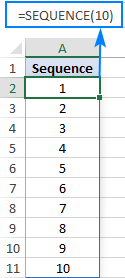To make a horizontal sequence, set the rows argument to 1 (or omit it) and define the number of columns, 8 in our case:

`=SEQUENCE(1,8)`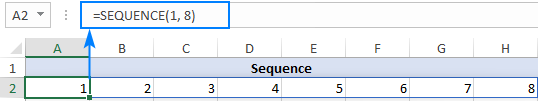If you'd like to fill a range of cells with sequential numbers, then define both the rows and columns arguments. For instance, to populate 5 rows and 3 columns, you'd use this formula:

`=SEQUENCE(5,3)`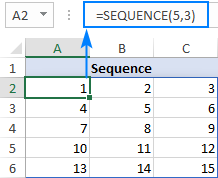To start with a specific number, say 100, supply that number in the 3rd argument:

`=SEQUENCE(5,3,100)`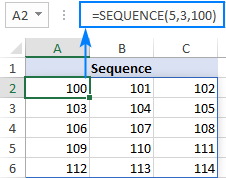To generate a list of numbers with a specific increment step, define the step in the 4th argument, 10 in our case:

`=SEQUENCE(5,3,100,10)`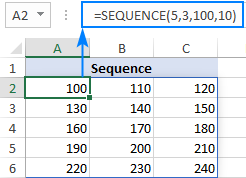Translated into plain English, our complete formula reads as follows:### SEQUENCE function - things to remember

To efficiently do a sequence of numbers in Excel, please remember these 4 simple facts:

• The SEQUENCE function is only available with Microsoft 365 subscriptions. In Excel 2019, Excel 2016 and earlier versions, it does not work since those versions do not support dynamic arrays.
• If the array of sequential numbers is the final result, Excel outputs all the numbers automatically in a so-called spill range. So, be sure you have enough empty cells down and to the right of the cell where you enter the formula, otherwise a #SPILL error will occur.
• The resulting array can be one-dimensional or two-dimensional, depending on how you configure the rows and columns arguments.
• Any optional argument that is not set defaults to 1.

## How to create a number sequence in Excel - formula examples

Though the basic SEQUENCE formula does not look very exciting, when combined with other functions, it takes on a whole new level of usefulness.

### Make a decreasing (descending) sequence in Excel

To generate a descending sequential series, such that each subsequent value is less than the preceding one, supply a negative number for the step argument.

For example, to create a list of numbers starting at 10 and decreasing by 1, use this formula:

`=SEQUENCE(10, 1, 10, -1)`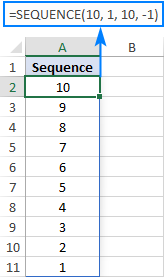### Force a two-dimensional sequence to move vertically top to bottom

When populating a range of cells with sequential numbers, by default, the series always goes horizontally across the first row and then down to the next row, just like reading a book from left to right. To get it to propagate vertically, i.e. top to bottom across the first column and then right to the next column, nest SEQUENCE in the TRANSPOSE function. Please note that TRANSPOSE swaps rows and columns, so you should specify them in the reverse order:

TRANSPOSE(SEQUENCE(columns, rows, start, step))

For example, to fill 5 rows and 3 columns with sequential numbers starting at 100 and incremented by 10, the formula takes this form:

`=TRANSPOSE(SEQUENCE(3, 5, 100, 10))`

To better understand the approach, please have a look at the screenshot below. Here, we input all the parameters in separate cells (E1:E4) and create 2 sequences with the below formulas. Please pay attention rows and columns are supplied in different order!

Sequence that moves vertically top to bottom (row-wise):

`=TRANSPOSE(SEQUENCE(E2, E1, E3, E4))`

Regular sequence that moves horizontally left to right (column-wise):

`=SEQUENCE(E1, E2, E3, E4)`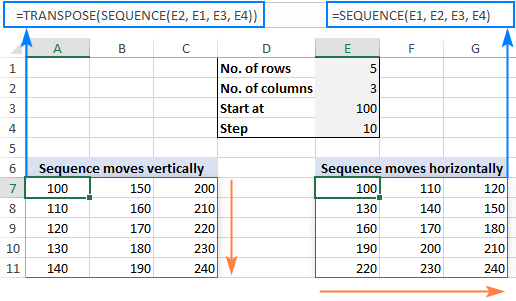### Create a sequence of Roman numbers

Need a Roman number sequence for some task, or just for fun? That's easy! Build a regular SEQUENCE formula and warp it in the ROMAN function. For example:

`=ROMAN(SEQUENCE(B1, B2, B3, B4))`

Where B1 is the number of rows, B2 is the number of columns, B3 is the start number and B4 is the step.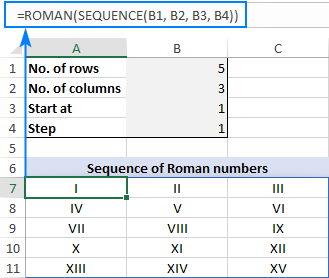### Generate an increasing or decreasing sequence of random numbers

As you probably know, in Excel 365 there is a special function for generating random numbers, RANDARRAY, which we discussed a few articles ago. This function can do a lot of useful things, but in our case it cannot help. To generate either an ascending or descending series of random whole numbers, we'll be needing the good old RANDBETWEEN function for the step argument of SEQUENCE.

For example, to create a series of increasing random numbers that spills in as many rows and columns as specified in B1 and B2, respectively, and start at the integer in B3, the formula goes as follows:

`=SEQUENCE(B1, B2, B3, RANDBETWEEN(1, 10))`

Depending on whether you want a smaller or bigger step, supply a lower or higher number for the second argument of RANDBETWEEN.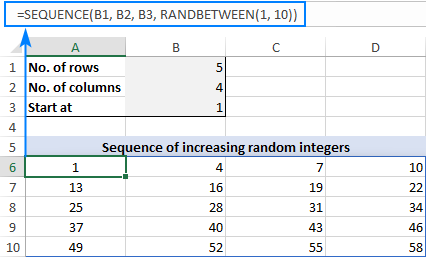To make a sequence of decreasing random numbers, the step should be negative, so you put the minus sign before the RANDBETWEEN function:

`=SEQUENCE(B1, B2, B3, -RANDBETWEEN(1, 10))`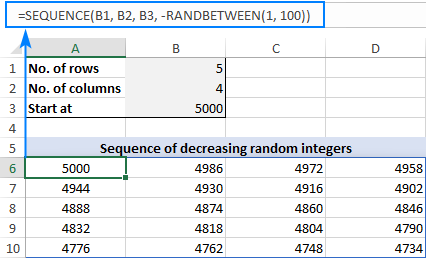Note. Because the Excel RANDBETWEEN function is volatile, it will generate new random values with every change in your worksheet. As the result, your sequence of random numbers will be continuously changing. To prevent this from happening, you can use Excel's Paste Special > Values feature to replace formulas with values.

## Excel SEQUENCE function missing

Like any other dynamic array function, SEQUENCE is only available in the latest builds of Excel 365 that support dynamic arrays. Therefore, you won't find any of those functions in pre-dynamic Excel such as Excel 2019, Excel 2016, and lower.

That's how to create sequence in Excel 365 with formulas. I hope the examples were both useful and fun. Anyway, thank you for reading and hope to see you on our blog next week!